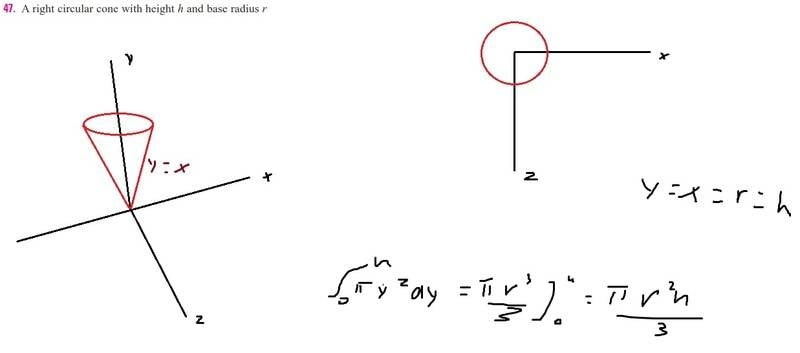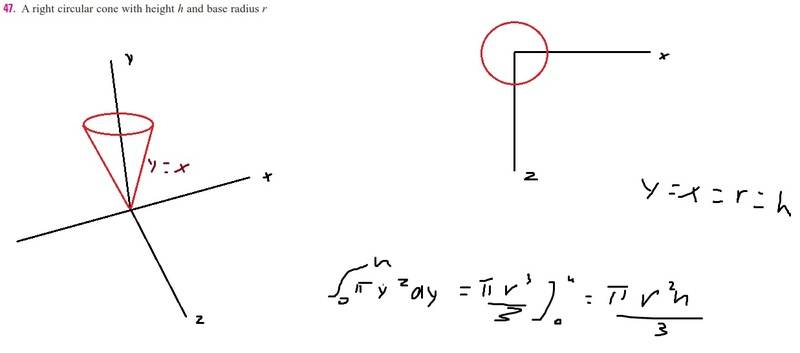# Volume of right circular cone

## Homework Statement

Find the volume of a right circular cone with height h and base radius r.

## The Attempt at a SolutionI've chosen the case where y = x = r = h. Then, I solve the integral in the image above and I got the book's answer. But is it actually correct?

LCKurtz
Homework Helper
Gold Member

## Homework Statement

Find the volume of a right circular cone with height h and base radius r.

## The Attempt at a SolutionI've chosen the case where y = x = r = h. Then, I solve the integral in the image above and I got the book's answer. But is it actually correct?
No. y and x are not constant and not equal to h.

But I've considered several cylinders along y axis from the origin to h and each of them has radius r = x. What is the problem here?

LCKurtz
Homework Helper
Gold Member
You are given ##r## is the radius of the base of the cone. You can't use it as a variable radius. And you can't assume ##y=x## making it a 45 degree cone. That isn't given.

SO what can I do to find the volume?

LCKurtz
Homework Helper
Gold Member
I would suggest the method of disks. Calculate the volume of a disc of thickness dy located at some y between 0 and h. You will need the equation of the straight line with the correct slope for the side to figure out the variable radius of the disc.

vela
Staff Emeritus
Homework Helper
But I've considered several cylinders along y axis from the origin to h and each of them has radius r = x. What is the problem here?
The problem is you're being incredibly sloppy with the notation, your reasoning is horribly off, or both.

##r## and ##h## represent dimensions of the cone. So you could assume ##r=h## and get a result, but that result is only good for when ##r=h## (in which case, you don't really need two variables). You can't now claim the expression you derived is good for any right cone with arbitrary dimensions.

When you write something like "y=x=r=h", it doesn't really make sense. What do ##y## and ##x## represent here? If we take seriously that ##y=r## and ##x=r##, they're constants because ##r## is a constant (the radius of the base), so how can you integrate over either variable?

I would suggest the method of disks. Calculate the volume of a disc of thickness dy located at some y between 0 and h. You will need the equation of the straight line with the correct slope for the side to figure out the variable radius of the disc.

Use this equation to work out the volume

Ok, I will do that

The problem is you're being incredibly sloppy with the notation, your reasoning is horribly off, or both.

You can't now claim the expression you derived is good for any right cone with arbitrary dimensions.

So a more "correct" way would be to integrate πy² over the interval [0, h], where y² = x² is the radius of each cylinder with width dy? The result would be πh³ / 3. But if I've chosen f(x) = y = x, then h would be equal to r (radius of the cone) in the end of the interval.

LCKurtz
Homework Helper
Gold Member
Ok, I will do that

So a more "correct" way would be to integrate πy² over the interval [0, h], where y² = x² is the radius of each cylinder with width dy? The result would be πh³ / 3. But if I've chosen f(x) = y = x, then h would be equal to r (radius of the cone) in the end of the interval.

As we have pointed out, ##y\ne x##. The radius of the disc is the ##x## value at that ##y##. You need the equation of the straight line representing the side to get ##x## in terms of ##y##.

•kent davidge